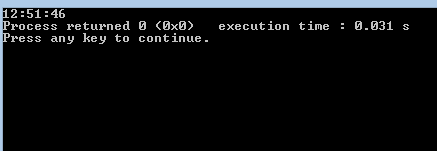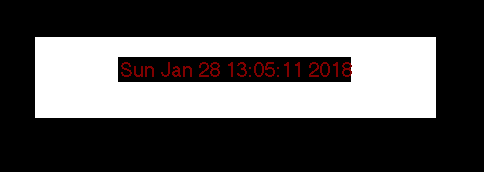Open In App

# C program to print digital clock with current time

The time.h header defines four variable types, two macro and various functions for manipulating date and time. A brief description of the variable types defined in the header time.h is as :

• size_t : This is the unsigned integral type and is the result of the sizeof keyword.
• clock_t : This is a type suitable for storing the processor time.
• time_t is : This is a type suitable for storing the calendar time.
• struct tm : This is a structure used to hold the time and date.

Below is the implementation of digital clock. On executing the program, the output window will show the time when the program was executed.

## C

 `// C implementation of digital clock``#include ``#include ` `// driver code``int` `main()``{``    ``time_t` `s, val = 1;``    ``struct` `tm``* current_time;` `    ``// time in seconds``    ``s = ``time``(NULL);` `    ``// to get current time``    ``current_time = ``localtime``(&s);` `    ``// print time in minutes,``    ``// hours and seconds``    ``printf``(``"%02d:%02d:%02d"``,``           ``current_time->tm_hour,``           ``current_time->tm_min,``           ``current_time->tm_sec);` `    ``return` `0;``}`

Output :Below is the C program to show the current time inside a rectangular bar. The output window shows the day, month, date, current time and year.

## C

 `// C program to print digital``// clock using graphics``#include ``#include ` `// driver code``int` `main()``{``    ``// DETECT is a macro defined in``    ``// "graphics.h" header file``    ``int` `dt = DETECT, gmode, midx, midy;``    ``long` `current_time;``    ``char` `strftime``;` `    ``// initialize graphic mode``    ``initgraph(&dt, &gmode, ``""``);` `    ``// to find mid value in horizontal``    ``// and vertical axis``    ``midx = getmaxx() / 2;``    ``midy = getmaxy() / 2;` `    ``// set current colour to white``    ``setcolor(WHITE);` `    ``// make a rectangular box in``    ``// the middle of screen``    ``rectangle(midx - 200, midy - 40, midx + 200,``                                     ``midy + 40);` `    ``// fill rectangle with white color``    ``floodfill(midx, midy, WHITE);``    ``while` `(!kbhit()) {` `        ``// get current time in seconds``        ``current_time = ``time``(NULL);` `        ``// store time in string``        ``strcpy``(``strftime``, ``ctime``(¤t_time));` `        ``// set color of text to red``        ``setcolor(RED);` `        ``// set the text justification``        ``settextjustify(CENTER_TEXT, CENTER_TEXT);` `        ``// to set styling to text``        ``settextstyle(SANS_SERIF_FONT, HORIZ_DIR, 3);` `        ``// locate position to write``        ``moveto(midx, midy);` `        ``// print current time``        ``outtext(``strftime``);``    ``}``    ``getch();` `    ``// deallocate memory for graph``    ``closegraph();``}`

Output :References :
cprogramforbeginners